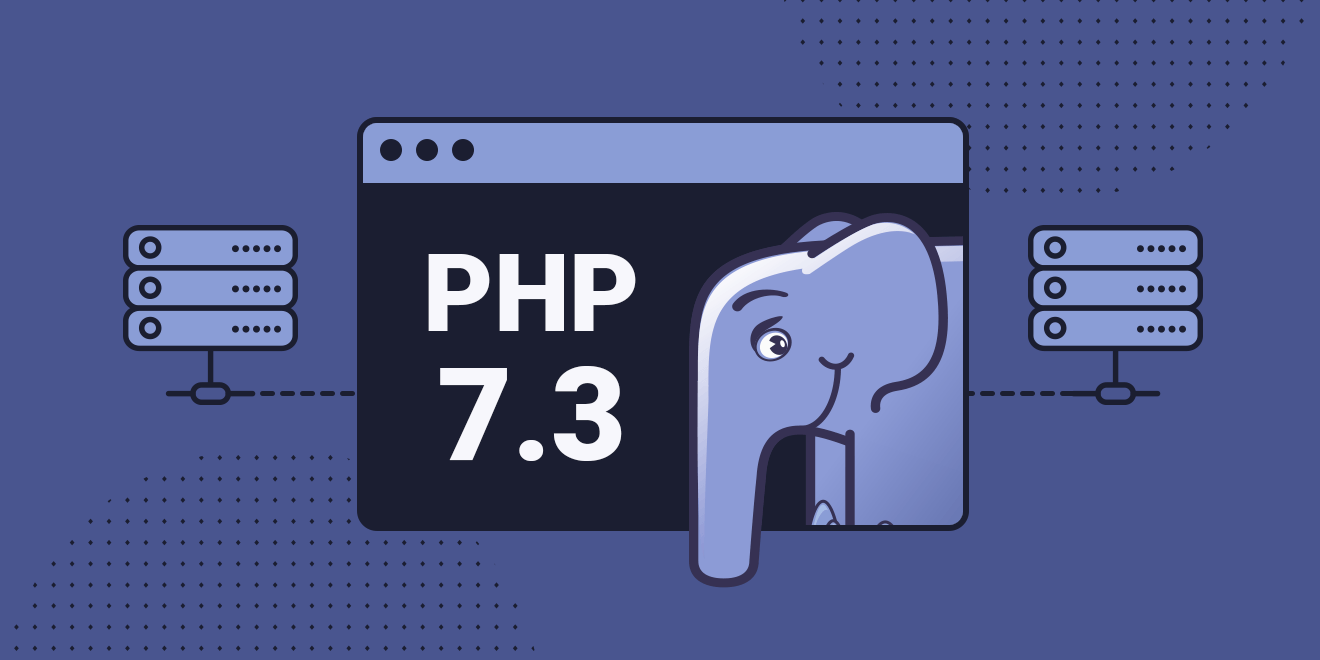PHP 目前依旧是其它脚本语言强劲的竞争对手，这主要归功于其核心维护团队的快速更新。

PHP 最近实现了又一个里程碑 —— 发布 PHP 7.3。新版本带来了一些急需的更新。

• 灵活的 Heredoc 和 Nowdoc 语法
• 函数调用时允许尾随逗号
• JSON_THROW_ON_ERROR
• PCRE2 迁移
• list() 分配参考
• is_countable 函数
• array_key_first(), array_key_last()
• Argon2 密码哈希增强功能
• 弃用和删除 image2wbmp()
• 弃用和删除不区分大小写的常量
• FPM 更新
• 改进 Windows 下的文件删除

## 灵活的 Heredoc 和 Nowdoc 语法

HeredocNowdoc 语法能够在使用多行长字符串时起到很大帮助。它要求结束标识符应当为出现在新行的首个字符串。

``````// 除了这样：

\$query = <<<SQL

SELECT *

FROM `table`

WHERE `column` = true;

SQL;

// 这样也可以：

\$query = <<<SQL

SELECT *

FROM `table`

WHERE `column` = true;

SQL;``````

1. 闭合标识符前支持缩进
2. 闭合标识符后不再强制换行

## 函数调用中允许尾部逗号

``````use Foo\Bar\{
Foo,
Bar,
};

\$foo = [
'foo',
'bar',
];``````

``````unset(
\$foo,
\$bar,
\$baz,
);``````

``````echo \$twig->render(
'index.html',
compact(
'title',
'body',
)
);``````

``````\$newArray = array_merge(
\$arrayOne,
\$arrayTwo,
['foo', 'bar'],
);``````

``````class Foo
{
public function __construct(...\$args) {
//
}

public function bar(...\$args) {
//
}

public function __invoke(...\$args) {
//
}
}

\$foo = new Foo(
'constructor',
'bar',
);

\$foo->bar(
'method',
'bar',
);

\$foo(
'invoke',
'bar',
);``````

## JSON_THROW_ON_ERROR

``````json_encode(\$data, JSON_THROW_ON_ERROR);

json_decode("invalid json", null, 512, JSON_THROW_ON_ERROR);

// 抛出 JsonException 异常``````

## 升级 PCRE2

PHP 使用 PCRE 作为正则表达式引擎。但从 PHP 7.3 开始，PCRE2 将作为新的正则引擎大显身手。所以，你需要将现有的正则表达式迁移到符合 PCRE2 的规则。这些规则比以前更具侵入性。请看以下实例：

``preg_match('/[\w-.]+/', '');``

• 相对后向引用 `\g{+2}`（等效于已存在的 `\g{-2}`
• PCRE2 版本检查 `(?(VERSION>=x)...)`
• `(*NOTEMPTY)``(*NOTEMPTY_ATSTART)` 告知引擎勿返回空匹配
• `(*NO_JIT)` 禁用 JIT 优化
• `(*LIMIT_HEAP=d)` 限制堆大小为 `d` KB
• `(*LIMIT_DEPTH=d)` 设置回溯深度限制为 `d`
• `(*LIMIT_MATCH=d)` 设置匹配数量限制为 `d`

## list() 赋值引用

PHP 中的 list() 现在可以赋值给引用，在当前版本中 list() 中赋值不能使用引用，在 PHP 7.3 中将允许使用引用，新改进的语法如下：

``````\$array = [1, 2];
list(\$a, &\$b) = \$array;``````

``````\$array = [1, 2];
\$a = \$array;
\$b =& \$array;``````

``````\$array = [[1, 2], [3, 4]];
foreach (\$array as list(&\$a, \$b)) {
\$a = 7;
}
var_dump(\$array);``````

## is_countable 函数

``````if (is_array(\$foo) || \$foo instanceof Countable) {
// \$foo 是可数的
}``````

``````if (is_countable(\$foo)) {
// \$foo 是可数的
}``````

## array_key_first(), array_key_last()

• `\$key = array_key_first(\$array);` 获取数组第一个元素的键名
• `\$key = array_key_last(\$array);` 获取数组最后一个元素的键名

``````// 关联数组的用法
\$array = ['a' => 1, 'b' => 2, 'c' => 3];

\$firstKey = array_key_first(\$array);
\$lastKey = array_key_last(\$array);

assert(\$firstKey === 'a');
assert(\$lastKey === 'c');

// 索引数组的用法
\$array = [1 => 'a', 2 => 'b', 3 => 'c'];

\$firstKey = array_key_first(\$array);
\$lastKey = array_key_last(\$array);

assert(\$firstKey === 1);
assert(\$lastKey === 3);``````

## Argon2 和 Hash 密码加密性能增强

Argon2id现在是在paswword_ *函数中使用的推荐的Argon2变量。

``````具有自定义成员方法的名称的Argon2id与PASSWORD_ARGON2I的使用方法相同

``````\$hash = password_hash('password', PASSWORD_ARGON2ID);

## 废弃并移除大小写不敏感的常量

• 类常量始终为「大小写敏感」。
• 使用 `const` 关键字定义的全局常量始终为「大小写敏感」。注意此处仅仅是常量自身的名称，不包含命名空间名的部分，PHP 的命名空间始终为「大小写不敏感」。
• 使用 `define()` 函数定义的常量默认为「大小写敏感」。
• 使用 `define()` 函数并将第三个参数设为 `true` 定义的常量为「大小写不敏感」。

• In PHP 7.3: 废弃使用 `true` 作为 `define()` 的第三个参数。
• In PHP 7.3: 废弃使用与定义时的大小写不一致的名称，访问大小写不敏感的常量。`true``false` 以及 `null` 除外。

PHP 7.3 在建议在使用 cookies 时，增加同站点标志。这个 RFC 会影响4个系统函数。

``````bool setcookie(

string \$name

[, string \$value = ""

[, int \$expire = 0

[, string \$path = ""

[, string \$domain = ""

[, bool \$secure = false

[, bool \$httponly = false ]]]]]]

)

string \$name

[, string \$value = ""

[, int \$expire = 0

[, array \$options ]]]

)

// 两种方式均可.``````

## FPM 更新

FastCGI 进程管理器也进行了更新，现在提供了新的方式来记录 FPM 日志。

log_limit: 设置允许的日志长度，可以超过 1024 字符。

log_buffering: 允许不需要额外缓冲去操作日志。

decorate _workers_output: 当启用了 catch_workers_output 时，系统会去禁用渲染输出。